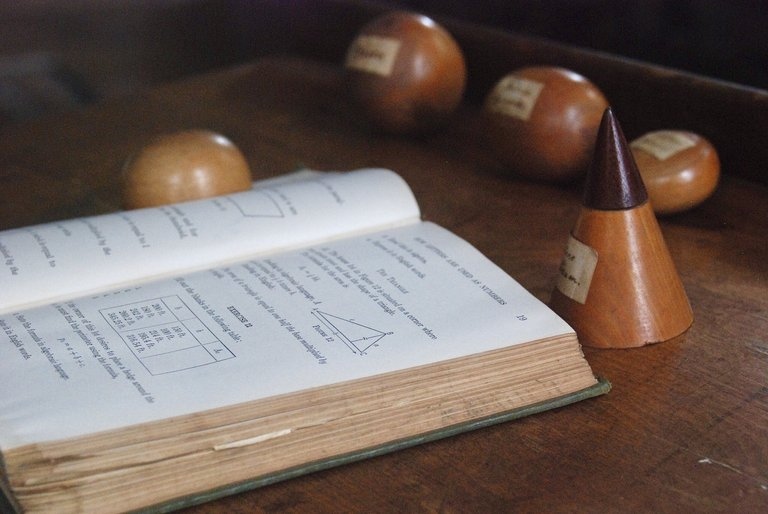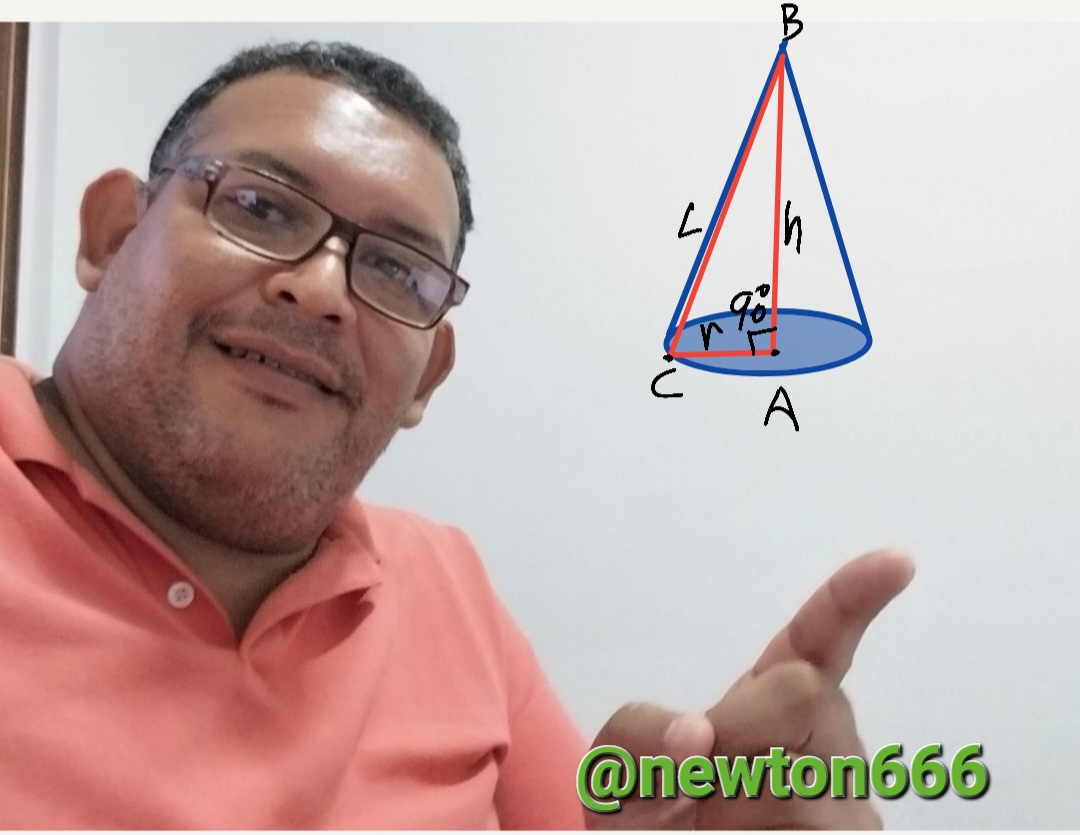# Electric charges under the geometry of a cone

in StemSocial2 months agoPixabay

Greetings, friend who loves science and is also good at physics, for this opportunity the singularity of knowledge is the creativity of tools that can be used to explain physical phenomena, but on this day the singularity is that it associates or links a geometric figure with electric charges for this occasion is a cone. The formula for the lateral surface area of a right cone is L.S.A. = π rl , where l is the pitch height of the cone.The cone is an intriguing three-dimensional geometric shape that is employed in many disciplines, including physics, engineering, and architecture. A circular base and a curved surface that converges towards a point known as the apex or vertex are the two characteristics that define the conical shape.

The strength and stability of a cone are greatly influenced by the loads that are applied to it. There are various sorts of loads that can be applied, including self-weight, wind, seismic, and use loads. It will be examined in this article how the loads must be arranged in a cone to ensure the stability and effectiveness of the building.

First and foremost, it's crucial to consider the cone's self-weight load. Based on the cone's dimensions and the density of the material used to build it, this load needs to be estimated. This load must be distributed equally throughout the building in order for the cone's curved surface to match the load's shape and the foundation to hold an acceptable amount of weight.

Another load that needs to be taken into account in a cone is the wind load. The wind speed, as well as the cone's size and shape, determine this load. The proper pressure coefficients must be used, and the force acting on the cone must be calculated, in order to calculate the wind load.This load must be distributed evenly over the entire surface of the cone to ensure its stability.

Another crucial loading to take into account in a cone is seismic loading. The location of the cone and the intensity in the vicinity of this determine this charge.

Let's say we have a cone with an even electric charge applied to its surface. We must take the following actions in order to determine the electric charge on the cone:

Determine the electric field at the cone's vertex:

Equation (1) gives the electric field at the vertex of a cone with uniformly distributed electric charge on its surface.

E = (Q / (4πε₀)) * (1 / h)

Where Q is the total electric charge on the cone, ε₀ is the electric permittivity of the vacuum, and h is the height of the cone.

Determine the electric flux through the cone's surface:
The equation: gives the electric flux through the cone's surface.

Φ = E * A

Where A is the cone's surface area and E the electric field at the cone's vertex.

Compute the cone's electric charge:

The electric charge on the cone is determined by dividing the electric flow through its surface by the vacuum's electric permittivity constant.

Q = Φ / ε₀

Consequently, we must take the following actions in order to compute the electric charge in a cone with an evenly distributed electric charge on its surface:

Using the formula E = (Q / (40))* (1 / h), get the electric field at the cone's vertex.

Using the formula = E * A, determine the amount of electricity flowing through the cone's surface.

Use the equation to determine the electric charge on the cone: Q = Φ / ε₀

Photo edited by my Samsung A23 phone

Bibliography Reference

Electronics Practices by Paul B. Zbar, ‎Albert Paul Malvino, ‎Michael A. Miller, 2003.

Physics for science and technology. II by Paul Allen Tipler, ‎Gene Mosca, 2004.

Electrostatics and Its Applications by A.D. Moore y A.F. Ciszek, 2012.

Geometric Algebra for Physicists by Chris Doran y Anthony Lasenby, 2003.

Geometry and Physics by J. Baez y J. Huerta, 2011.Sort:Thanks for your contribution to the STEMsocial community. Feel free to join us on discord to get to know the rest of us!

Please consider delegating to the @stemsocial account (85% of the curation rewards are returned).

Thanks for including @stemsocial as a beneficiary, which gives you stronger support.